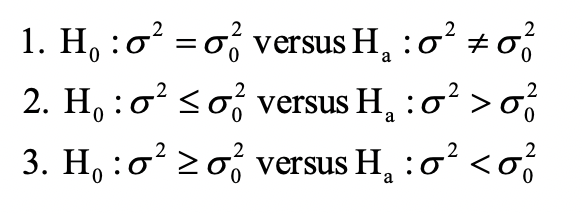# Hypothesis Tests Concerning Variances

Till now the tests we saw were related to population mean. We can also conduct hypothesis tests concerning the variance of the population.

There are two types of tests:

• Tests concerning the value of a single population variance
• Tests concerning the differences between two population variances

The hypothesis will be formed as follows:Where  is the population variance and  is the hypothesized value of the variance.

Get our Data Science for Finance Bundle for just $29$51.